# AP Chemistry : Reaction Types

## Example Questions

### Example Question #1 : Using Base Dissociation Constant (Kb)

A 1M solution of a monoprotic acid has a pH of 4.6. What is the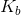value for the conjugate base of the acid?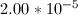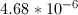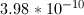Explanation:

In order to find the base dissociation constant for the conjugate base, we can start by finding the acid dissociation constant for the acid. Since a 1M solution of the acid has a pH of 4.6, we can find the proton concentration of the solution.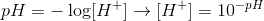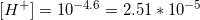Since the acid is monoprotic, we can set the following equilibrium expression equal to its acid dissociation constant.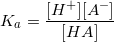We can see that, since the acid is monoprotic, the concntration of protons will be equal to the concentration of the acid anion. The final concentration of the acid molecule will be equal to the initial concentration, minus the amount of protons formed. Using these values, we can solve for the equilibrium constant for the acid.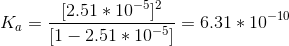Now that we have the acid dissociation constant, we can find the conjugate base's dissociation constant by setting the product of the two values equal to the autoionization of water.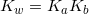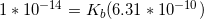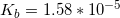### Example Question #1 : Strong And Weak Acids And Bases

Would H2SO4 or HNO3 produce a more acidic solution?

HNO3 since it has a higher pKa

H2SO4 since it has a lower pKa

HNO3 since it has a lower pKa

H2SO4 since it has a higher pKa

H2SO4 since it has a lower pKa

Explanation:

Both are strong acids, but H2SO4 is bivalent, realeasing 2 protons for each molecule dissolved in solution. Further, a more acidic solution would have a lower pKa.

### Example Question #1 : Strong And Weak Acids And Bases

Which of the following species will not be present in an aqueous solution of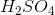?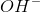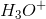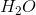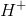All of these will be present in the solutionExplanation:

The hydroxide ion (OH–) is a strong base and therefore would not be present in an acidic solution. Protons, the hydronium ion, and water will all be present in relatively large amounts within the solution.

### Example Question #2 : Strong And Weak Acids And Bases

Which of the following anions is the strongest base?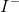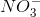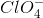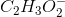Explanation:

Weaker acids dissociate less in water and therefore, reverse reaction is favored in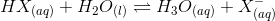. This indicates that the weaker the acid, the stronger its conjugate base.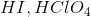, and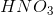are all strong acids. Their conjugates are weak bases.has the weakest conjugate acid among the all the choices, so it is the strongest base.

### Example Question #1 : Titrations And Indicators

A buffer using acetic acid (pKa=4.76) is titrated with NaOH. What is the pH at half the equivalence point?

7.00

4.76

9.52

2.38

12.36

4.76

Explanation:

The pH at half the equivalence point is equal to the pKa of the acid.

### Example Question #1 : Titrations And Indicators

At what pH does the equivalence point lie for a strong-acid / strong-base titration?

It is impossible to determine

Slightly basic

7

Slightly acidic

7

Explanation:

The equivalence point for a strong-acid / strong-base titration will be at neutral pH, 7. This is because each equivalent of the acid will neutralize each equivalent of the base, and you will be left with a neutral solution.

### Example Question #1 : Titrations And Indicators

At what pH does the equivalence point lie for a strong-acid / weak-base titration?

7

It is impossible to determine

Slightly basic

Slightly acidic

Slightly acidic

Explanation:

The equivalence point for a strong-acid / weak-base titration will be at a slightly acidic pH. This is because the acid is stronger and dissociates to a greater degree, while the base is not quite as strong, so doesn't dissociate to a large enough extent to neutralize each equivalent of the acid.

### Example Question #1 : Titrations And Indicators

At what pH does the equivalence point lie for a weak acid-strong base titration?

7

It is impossible to determine

Slightly basic

Slightly acidic

Slightly basic

Explanation:

The equivalence point for a weak-acid / strong-base titration will be at a slightly basic pH. This is because the base is stronger and dissociates to a greater degree, while the acid is not quite and strong and doesn't dissociate to a large enough extent to neutralize each equivalent of the base.

### Example Question #2 : Titrations And Indicators

You are given 500 mL of a HCl solution of unknown concentration and you titrate is with 0.0540 M NaOH. It takes 32.1 mL of the NaOH solution to reach your end point. What is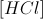of your original solution?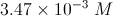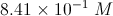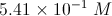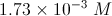Explanation:

First, let us write out the reaction that occurs: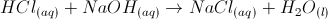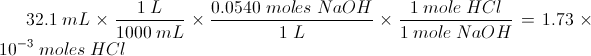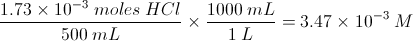### Example Question #1 : Titrations And Indicators

A titration is a drop-by-drop mixing of an acid and a base in order to determine the concentration of an unknown solution, via addition of a solution with known concentration. A titration curve can be graphed showing the relationship between the mixture pH and the amount of known solution added.

What would the titration curve look like for a strong base being titrated with a strong acid?

An increasing sigmoidal curve

A decreasing sigmoidal curve

A positively sloped line

A negatively sloped line

A decreasing sigmoidal curve

Explanation:

There are two things to consider here.

1. Since the solution is originally a strong base, the pH will be originally elevated. As a strong acid is added to the solution, the pH will decrease. As a result, the titration curve will be decreasing as the volume of titrant increases.

2. The titration curve will never be a straight line. Eventually, the strong acid will be much larger in volume than the original base; however, the pH will eventually even out at the pH of the added titrant.

Since we are titrating a strong base with a strong acid, the titration curve will be represented by a decreasing sigmoidal curve.

### All AP Chemistry Resources